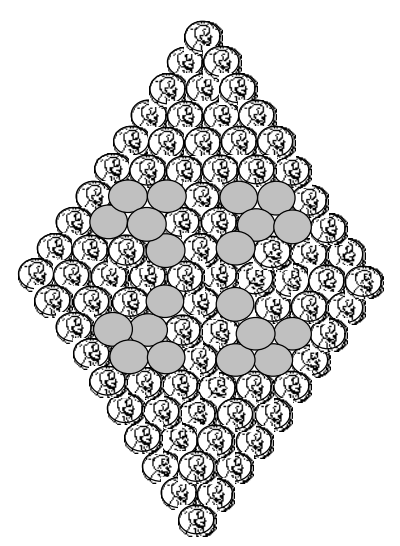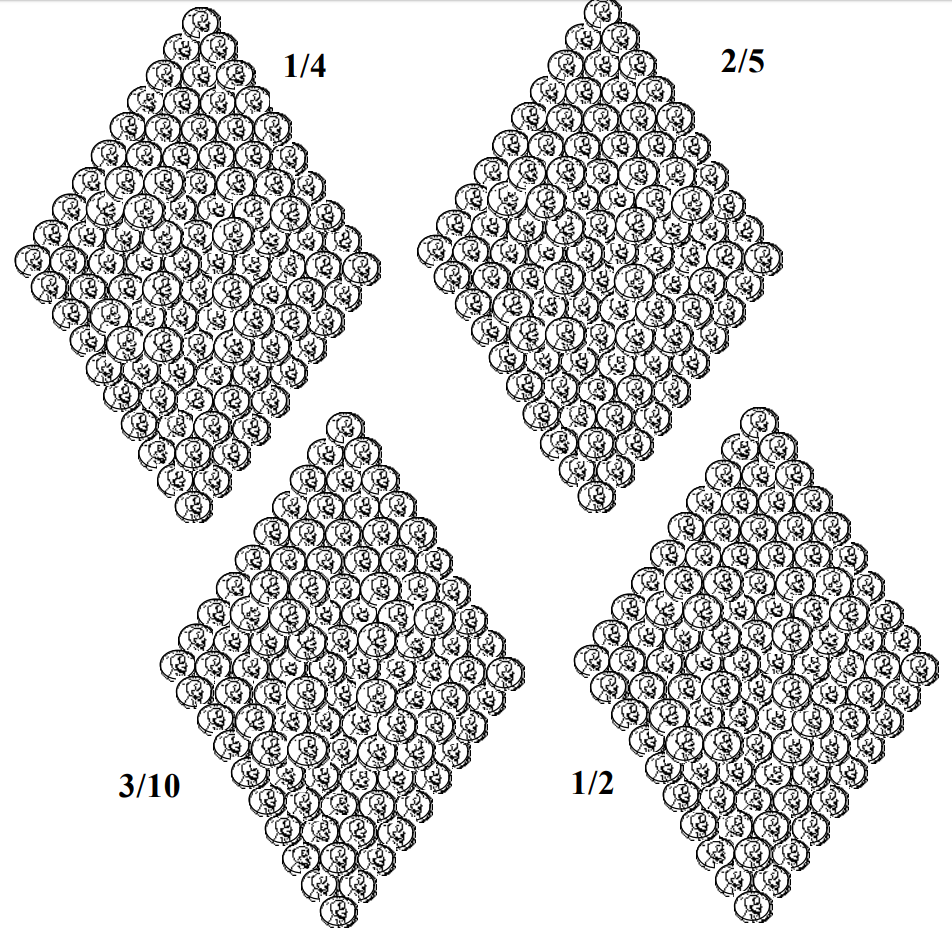Subject:
Mathematics

Topics: Percents
Common Core State Standard: 6.RP.3c, 6.RP.3d,
Concepts:

· Percent

Knowledge and Skills:

· Can convert between percent notation and fraction notation.

Lesson:

Procedure: This activity is best done by students working individually.

Hand out the two activity sheets to each student. Discuss the first sheet and ensure all students understand the key elements of the exercise: that there are 100 pennies in the shape, that a certain fraction of those pennies have been shaded to make a pattern, and they are to compute the percent that is shaded.

Have each student determine what percent is shaded in the given pattern, and discuss their results as a class. Some may determine the percent by simply counting the pennies, and others may convert the fraction “1/5” to a percent. Either method is fine.

Next have students create their own percent patterns using the four templates on the second sheet, in such a way that the given fractions of pennies are shaded in each case.

As an alternative, you may have each student create a pattern for a fraction that they choose or you assign. (To avoid the need to shade partial pennies and compute with percents which themselves have fractional elements, use fractions in which the denominator is a factor of “100”.)

### Penny Percent Patterns

There are one hundred pennies in the shape below.

1/5 of them have been shaded to make a pattern.